Courses

# Subjective Type Questions: Equilibrium- 2 | JEE Advanced Notes | EduRev

## JEE : Subjective Type Questions: Equilibrium- 2 | JEE Advanced Notes | EduRev

The document Subjective Type Questions: Equilibrium- 2 | JEE Advanced Notes | EduRev is a part of the JEE Course Class 11 Chemistry 35 Years JEE Mains &Advance Past yr Paper.
All you need of JEE at this link: JEE

Q.21. A 40.0 ml solution of weak base, BOH is titrated with 0.1N HCl solution. The pH of the solution is found to be 10.04 and 9.14 after adding 5.0 ml and 20.0 ml of the acid respectively. Find out the dissociation constant of the base.

Ans. 1.828 × 10–5

Solution. Case I. Write the concerned chemical reaction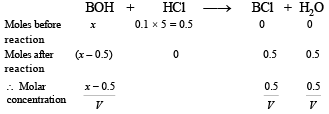Since the solution represents a basic buffer, following Hendersen equation can be applied.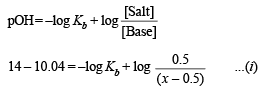Case II.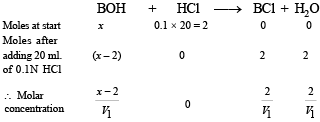Again the solution is acting as basic buffer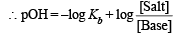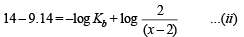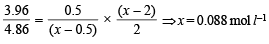Substituting x in (i) and solving for Kb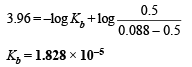Q.22. The solubility product (Ksp) of Ca(OH)2 at 25ºC is 4.42 × 10–5. A 500 ml. of saturated solution of Ca(OH)2 is mixed with equal volume of 0.4 M NaOH. How much Ca(OH)2 in milligrams is precipitated?

Ans. 743.3 mg

Solution. Let the solubility of Ca(OH)2 in pure water = S moles/litre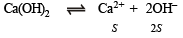Then  Ksp = [Ca2+] [OH]2

4.42 × 10–5 = S × (2S)2; 4.42 × 10–5 = 4S3
S = 2.224 × 10–2 = 0.0223 moles litre–1

∴ No. of moles of Ca2+ ions in 500 ml. of solution = λ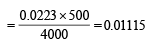NOTE THIS STEP : Now when 500 ml. of saturated solution is mixed with 500 ml of 0.4M NaOH, the resultant volume is 1000 ml. The molarity of OH– ions in the resultant solution would therefore be 0.2 M.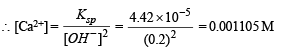Thus, No. of moles of Ca2+ or Ca(OH)2 precipitated = 0.01115 – 0.001105 = 0.010045

Mass of Ca(OH)2 precipitated

= 0.010045 × 74 = 0.7433 g = 743.3 mg

[mole wt. of Ca(OH)2 = 74]

Q.23. 0.15 mole of CO taken in a 2.5 l flask is maintained at 750 K along with a catalyst so that the following reaction can take place :Hydrogen is introduced until the total pressure of the system is 8.5 atmosphere at equilibrium and 0.08 mole of methanol is formed. Calculate (i) Kp and Kc and (ii) the final pressure if the same amount of CO and H2 as before are used, but with no catalyst so that the reaction does not take place.

Ans. (i) 0.05 atm–2, 187.85 mol–2 l2, (ii) 12.438 atm

Solution. (i)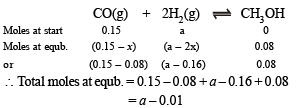Total moles at equilibrium can also be calculated from the following relation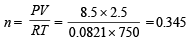∴ 0.345 = a – 0.01 [Comparing (i) and (ii)]

or  a   =  0.355

Thus, Moles of CO at equilibrium = 0.15 – 0.08 = 0.07

Moles of H2 at equilibrium = 0.355 – 0.16 = 0.195

Moles of CH3OH at equilibrium = 0.08

Substituting the values in the relation,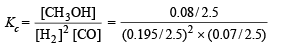=  187.85 mole–2 litre2       [∵ V = 2.5 L]

Calculation of Kp

Kp = Kc (RT)Δn = 187.85 × (0.0821 × 750)–2 = 0.05 atm–2

[∵ Δn = –2]

(ii) Calculation of final pressure when there is no reaction

Moles of CO = 0.15; Moles of H2 = 0.355

∴ Total moles = 0.15 + 0.355 = 0.505

PV = nRT

P × 2.5 = 0.505 × 0.0821 × 750 ⇒ P = 12.438 atm.

Q.24. The pH of blood stream is maintained by a proper balance of H2CO3 and NaHCO3 concentrations. What volume of 5M NaHCO3 solution should be mixed with a 10 ml sample of blood which is 2M in H2CO3 in order to maintain a pH of 7.4 ? Ka for H2CO3 in blood is 7.8 × 10–7

Ans. 78.36 ml

Solution. Volume of blood = 10 ml. (given)
[H2CO3] in blood = 2 M (given)
[NaHCO3] to be added = 5 M (given)
Let volume of NaHCO3 added in 10 ml blood = V ml

∴ [H2CO3] in blood mixture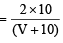[NaHCO3] in blood mixture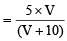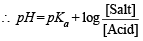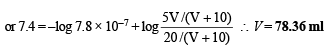Q.25. An aqueous solution of a metal bromide MBr2 (0.05M) is saturated with H2S. What is the minimum pH at which MS will precipitate?

Ksp for MS = 6.0 x 10 -21; concentration of saturated H2S = 0.1 M
K1 = 10–7 and K2 = 1.3 x 10–13 , for H2S.

Ans. 0.983

Solution.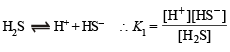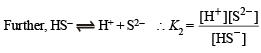Dissociation constant of H2S, K = K1 × K2

i.e.K = 1 × 10–7 × 1.3 × 10–13 = 1.3 × 10–20

Now we know that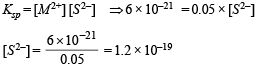Substituting the various values in the following relation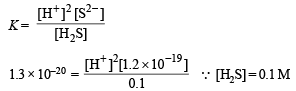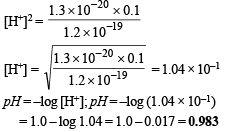Q.26. At temperature T, a compound AB2 (g) dissociates according to the reaction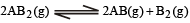with a degree of dissociation x which is small compared with unity. Deduce the expression for x in terms of the equilibrium constant Kp and the total pressure, P.

Ans.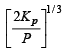Solution.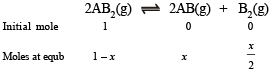Total moles at equb.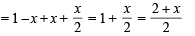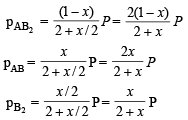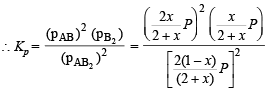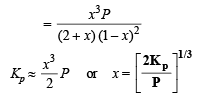Q.27. For the reaction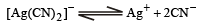the equilibrium constant, at 25°C , is 4.0 x 10 -19 . Calculate the silver ion concentration in a solution which was originally 0.10 molar in KCN and 0.03 molar in AgNO3.

Ans. 7.5 × 10–18 M

Solution. TIPS/Formulae : Consider common ion effect

Conc. of Ag+ ions =  Conc. of AgNO3 = 0.03 M

Most of these Ag+ ions will be present in the form of [Ag(CN)2].

0.03 M AgNO3 requires 2 × 0.03 M

= 0.06 M CN to form [Ag(CN)2]

∴ Conc. of free CN at equilibrium will be 0.1 – 0.06 = 0.04 M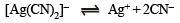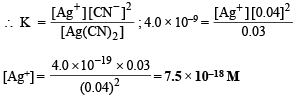Q.28. Calculate the pH of an aqueous solution of 1.0 M ammonium formate assuming complete dissociation.

(pKa of formic acid = 3.8 and pKb of ammonia = 4.8.)

Ans. 6.5

Solution. For ammonium formate which is a salt of weak acid with weak base, we know that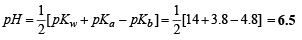Q.29. What is the pH of a 0.50 M aqueous NaCN solution? pKb of CN is 4.70.

Ans. 11.5

Solution.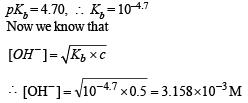Now we know that
pOH  = –log[OH–]
pOH = –log 3.158 × 10–3 = 2.5
or,  pH  = 14 – 2.5 = 11.5

Q.30. A sample of AgCl was tr eated with 5.0 0 mL of 1.5 M Na2CO3 solution to give Ag2CO3. The remaining solution contained 0.0026 g of Cl per litre. Calculate the solubility product of AgCl (Ksp(Ag2CO3) = 8.2 × 10–12).

Ans. 1.71 × 10–10

Solution. The concerned chemical reaction is

2AgCl + Na2CO3 → Ag2CO+ 2 NaCl

Calculation of [Ag+] left in the solution :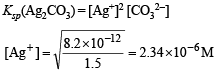Concentration of Cl– left = 0.0026 g/l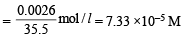∴ Ksp(AgCl) = [Ag+] [Cl] = (2.34 × 10–6) (7.33 × 10–5

= 1.71 × 10–10

Q.31. An acid type in dicator, HIn differ s in colour fr om its conjugate base (In). The human eye is sensitive to colour differences only when the ratio[In]/[HIn] is greater than 10 or smaller than. 0.1. What should be the minimum change in the pH of the solution to observe a complete colour change (Ka=1.0×10–5)?

Ans. 2

Solution. Given Ka = 1 × 10–5

∴ pKa = 5

The two conditions when colour indicator will be visible are derived by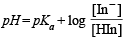(i) pH = 5 + log 10 = 6

(ii) pH = 5 + log 0.1 = 4

Thus minimum change in pH = 2

Q.32. Given :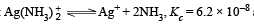and Ksp of AgCl = 1.8 × 10–10  at 298 K. If ammonia is added to a water solution containing excess of AgCl(s) only, calculate the concentration of the complex in 1.0 M aqueous ammonia.

Ans. 0.0538 M

Solution.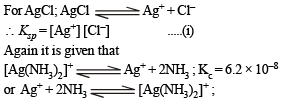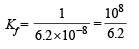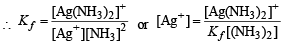NOTE THIS STEP : Since the formation constant of the complex is very high, most of the [Ag+] which dissolves must be converted into complex and each Ag+ dissolved also requires dissolution of Cl.

∴ [Cl] = [Ag (NH3)2]+ and let it be c M

Equation (i) becomes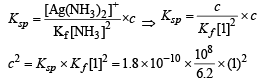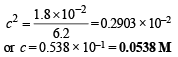Q.33. What will be the resultant pH when 200mL of an aqueous solution of HCl (pH = 2.0) is mixed with 300  mL of an aqueous solution of NaOH (pH = 12.0) ?

Ans. 11.3010

Solution.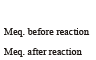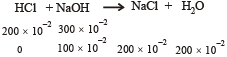pH of HCl = 2, pH of  NaOH = 12

∴ [HCl] = 10–2 M, ∴ [NaOH] = 10–2 M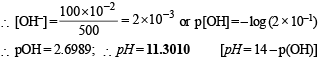Q.34. When 3.06 g of solid NH4HS is introduced into a two litre evacuated flask at 27° C, 30% of the solid decomposes into gaseous ammonia and hydrogen sulphide. (i) Calculate Kc and Kp for the reaction at 27°C. (ii) What would happen to the equilibrium when more solid NH4HS is introduced into the flask ?

Ans. 8.1 × 10–5 mol2 l–2, 4.90 × 10–2 atm2

Solution.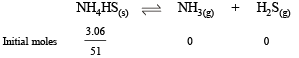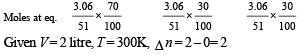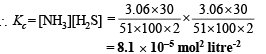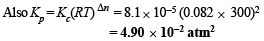NOTE : Addition of more NH4HS on this equilibrium will cause no effect because concentration of NH4HS is not involved in formula of Kp or Kc.

Q.35. The solubility of Pb(OH)2 in water is 6.7×10–6 M. Calculate the solubility of Pb(OH)2 in a buffer solution of  pH = 8.

Ans. 1.203 × 10–3 mol litre–1

Solution.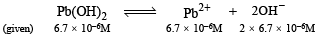∴ Ksp = [Pb2+][OH-]2 = (6.7 × 10– 6) (2 × 6.7 × 10– 6)2

= 1.203 ×10–15

The buffer solution pH = 8 (given)

∴ pOH = 6 or [OH] = 10–6

Thus in this buffer we have,   [Pb2+][OH]2 = 1.203 x 10–15

or  [Pb2+]  ×  [10–6]2 = 1.203 x 10–15

∴ [Pb2+]   =  1.203 x 10–3 mol litre–1

Q.36. The average concentration of SO2 in the atmosphere over a city on a certain day is 10 ppm, when the average temperature is 298 K. Given that the solubility of SO2 in water at 298 K is 1.3653 moles litre–1 and the pKa of H2SO3 is 1.92, estimate the pH of rain on that day.

Ans. 4.865

Solution. Amount of SO2 in atmosphere =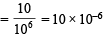Molar concentration of SO2 present in water = Amount of SO2 × Solubility of SO2 in water = 10 × 10–6 × 1.3653 mole L–1 = 1.3653 × 10–5 M

Writing the concerned chemical equation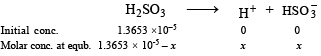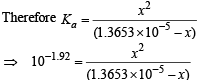(pKa = 1.92, ∴ Ka = 10–1.92)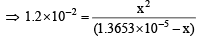x2 = 1.2 × 10–2 (1.3653 × 10-5 – x) On solving, x = 1.364 × 10–5

Therefore, pH = – log (1.364 × 10–5) = 4.865

Q.37. 500 mL of 0.2 M aqueous solution of acetic acid is mixed with 500 mL of 0.2 M HCl at 25°C.

(i) Calculate the degree of dissociation of acetic acid in the resulting solution and pH of the solution.
(ii) If 6 g of N aOH is a dded to th e above soluti on , determine the final pH. [Assume there is no change in volume on mixing; Ka of acetic acid is 1.75 × 10-5 mol L-1].

Ans. 1.75 × 10–4, 1, 4.75

Solution. (i) The volume being doubled by mixing the two solutions, the molarity of each component will be halved i.e.

[CH3COOH] = 0.1 M, [HCl] = 0.1 M.

NOTE :

HCl being a strong acid will remain completely ionised and hence H+ ion concentration furnished by it will be 0.1 M. This would exert common ion effect on the dissociation of acetic acid, (a weak acid.)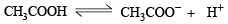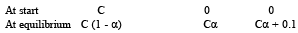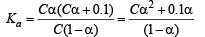Since α is very very small, Ca2 can be neglected and 1 – α can be taken as unity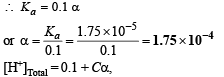Cα is negligible as compared to 0.1.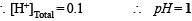(ii)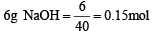0.1 mole of NaOH will be consumed by 0.1 mole of HCl.
Thus, 0.05 mole of NaOH will react with acetic acid according to the equation.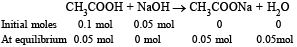Thus, solution of acetic acid and sodium acetate will become acidic buffer. So pH of the buffer will be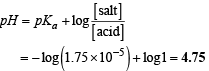Q.38. Match the following if the molecular weights of X, Y and Z are same.

 Boiling Point Kb X 100 0.63 V 27 0.53 z 253 0.98

Ans. x = 0.63, y = 0.53, z = 0.98

Solution. TIPS/Formulae :

Higher the value of dipole-dipole interaction higher is b.p.
Higher value of Kb of a solvent suggests larger polarity of solvent molecules which in turn leads to higher dipole – dipole interaction implies higher boiling point due to dipole – dipole interaction. Therefore, the correct order of Kb values of the three given solvents is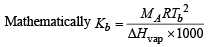or  Kb ∝Tb (b.pt.)

 Solvents Boiling point Kb values X 100°C 0.63 Y 27°C 0.53 Z 283°C 0.98
Offer running on EduRev: Apply code STAYHOME200 to get INR 200 off on our premium plan EduRev Infinity!

54 docs|30 tests

,

,

,

,

,

,

,

,

,

,

,

,

,

,

,

,

,

,

,

,

,

;